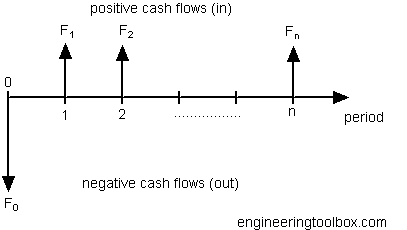Engineering ToolBox - Resources, Tools and Basic Information for Engineering and Design of Technical Applications!

# Perpetuities

## An asset providing a fixed cash flow.

A perpetuity is an asset that provides a never ending periodic fixed amount of cash flow.Endowments and trust funds and long lived assets like oil wells or gas fields can be regarded as a perpetuity.

The value of a perpetuity can be calculated as

P = F1 / (1 + i)1F2 / (1 + i)2 F3 / (1 + i)3 + ...... + Fn / (1 + i)n

= F / i                                    (1)

where

P = present value

F = F1 = F2  .. Fn  = the fixed amount of cash flow per period

i = discount rate of return (per time period)

### Example - The Value of a Company and Net Income

A company is able to deliver a net income every year of 100. Assuming that this is a perpetuity - a never ending income - the value of this cash flow (and the value of the company) with a discount rate of 10% (i = 0.10) can be calculated to

P = (100) / 0.10

= 1000

### Growing Perpetuity

If a cash flow grows in a constant rate the value of the perpetuity can be expressed as

P = F / (i - g)        (2)

where

g = growth rate of cash flow (per time period)

### Example - The Value of a Growth Company and Net Income

A growth company is able to deliver a net income first year of 200 with a net income growth rate the next years of 3% (g = 0.03). Assuming that this is a perpetuity - a never ending income - the value of this cash flow (and the value of the company) with a discount rate of 10% (i = 0.10) can be calculated to

P = (100) / (0.10 - 0.03)

= 1429

### Perpetuity Value Calculator

F - amount

i - discount rate (%)

g - growth rate (%)

## Related Topics

• Economics - Engineering economics - cash flow diagrams, present value, discount rates, internal rates of return - IRR, income taxes, inflation.

## Engineering ToolBox - SketchUp Extension - Online 3D modeling!

Add standard and customized parametric components - like flange beams, lumbers, piping, stairs and more - to your Sketchup model with the Engineering ToolBox - SketchUp Extension - enabled for use with the amazing, fun and free SketchUp Make and SketchUp Pro .Add the Engineering ToolBox extension to your SketchUp from the SketchUp Pro Sketchup Extension Warehouse!

Translate

## Privacy

We don't collect information from our users. Only emails and answers are saved in our archive. Cookies are only used in the browser to improve user experience.

Some of our calculators and applications let you save application data to your local computer. These applications will - due to browser restrictions - send data between your browser and our server. We don't save this data.

## Citation

• Engineering ToolBox, (2009). Perpetuities. [online] Available at: https://www.engineeringtoolbox.com/perpetuity-d_1497.html [Accessed Day Mo. Year].

Modify access date.

. .

#### Scientific Online Calculator3 30